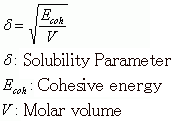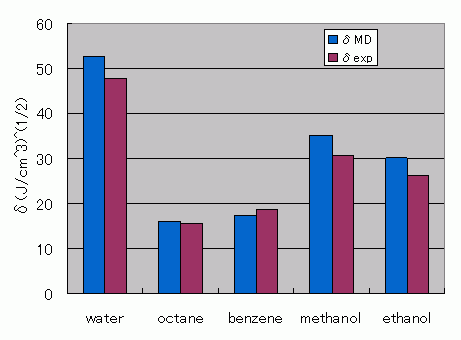Category
• Engine -
• COGNAC/VSOP
• Materials -
• Plastics, Rubbers/Elastomers, Solution

# Evaluation of Solubility Parameters Using MD

Using the full atomistic model to perform MD calculations, the solubility parameter (SP value, ƒÂ) has been evaluated for water, octane, benzene, ethanol, and methanol. Water calculations were made using the SPC-FW model. All other molecules were calculated using J-OCTA modeling (GAFF was used for the force-field values, and MO calculations were used for the charge values). The number of molecules was chosen such that the number of particles in the system would be approximately 700 particles. A single-composition system was created from each molecule. Boundary conditions were set to periodic boundary conditions, and the MD calculations were made using our solver VSOP.

After performing MD calculations on the NTP ensemble at 300 K and relaxing the system, further calculations were performed to obtain time series data for the evaluation of the SP. The J-OCTA DPD modeler function was used to compute the SP value. This procedure used the following formula to compute the solubility parameter, where the cohesive energy is obtained from the difference in the potential energy of the isolated chain in a pseudo vacuum and the potential energy of the bulk state.Figure 1. Comparison of SP Values Obtained from MD Calculations Against the Experimental Data

Table 1 Density and SP - Experimental Data and MD Estimations
ρMD
[g/cm^3]
δMD
[(J/cm^3)^(1/2)]
ρexp
[g/cm^3]
δexp[1,2]
[(J/cm^3)^(1/2)]
water 1.01 52.6 1.00 47.9
octane 0.69 16.0 0.70 15.6
benzene 0.85 17.4 0.88 18.6
methanol 0.83 35.0 0.79 29.7
ethanol 0.82 30.1 0.79 26.2
• Reference
•  A.Maiti and S. McGrother, J. Chem. Phys., 120, 3, 15 (2004)
•  Allan F. M. Barton, The CRC Handbook of Solubility Parameters and Other Cohesion Parameters, Second Edition CRC Press, (1991)